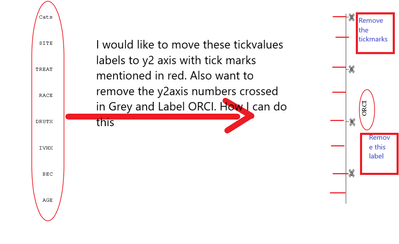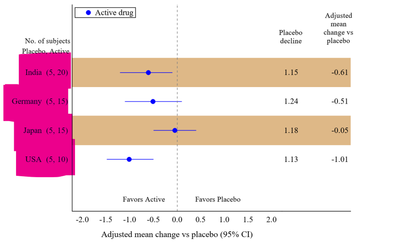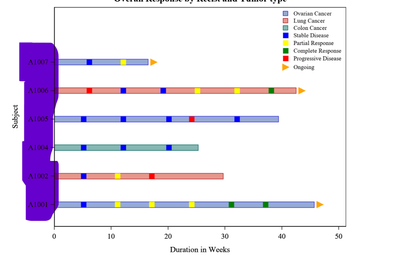## How to control Y2axis tick value labels using PROC TEMPLATE

I am trying to create a graph expecting with y2axis tick labels and  removing  y2axis label. How I can achieve it.  any suggestions? thank you.``````data orci;
input effect\$ OR LCL UCL;
cards;
AGE 1.05 1.01 1.09
BEC 1.00 0.98 1.02
IVHX 0.70 0.54 0.89
DRUTX 0.94 0.90 0.99
RACE 1.26 0.81 1.95
TREAT 1.54 1.04 2.27
SITE 1.13 0.74 1.72
;
RUN;
data orci1;

effect='Cats';
run;

data orci2;
set orci orci1;
orci=_n_;
xval=0;
run;

proc template;
define statgraph xx;
begingraph/BORDER=off;

layout overlay /  border=false walldisplay= none
yaxisopts=(reverse=false type=discrete display=none)
xaxisopts = (display=none offsetmin=0 offsetmax=.7);
entry  halign=center " " / pad=(right=40%) location=outside
valign=top textattrs=(family="Courier New" size=10pt);

scatterplot  x=xval y=orci / markercharacter=effect  yaxis=y2
markercharacterposition= left markercharacterattrs=(family="Courier New" size=10pt);

endlayout;
endgraph;
end;
run;

proc sgrender data=orci2 template="xx";
run;

``````

1 ACCEPTED SOLUTION

Accepted Solutions

## Re: How to control Y2axis tick value labels using PROC TEMPLATE

Your "text" are not axis labels at all from your shown example. They are marker labels.

One way to get the shown text as an axis label is a format for the actual variable making the axis with the desired values and associate the format with the variable in either the data or the Sgrender code.

Other is in the Yaxisopts or Y2axisopts for DISCRETE axis you can use a number of options such as TICKVALUELIST , TICKVALUEDISPLAYLIST and TICKVALUEFORMAT The lists are rather fixed or require dynamic values.

You control options on the Y2 axis with Y2axisopts .

This gets most of what I think you are asking for with the given demo data.

```proc format ;
value orci
1='AGE'
2='BEC'
3='IVHX'
4='DRUTX'
5='RACE'
6='TREAT'
7='SITE'
;
run;
proc template;
define statgraph xx;
begingraph/BORDER=off;

layout overlay /  border=false walldisplay= none
yaxisopts=(reverse=false type=discrete display=none )
y2axisopts=(type=discrete tickstyle=inside
label=" "
)
xaxisopts = (display=none offsetmin=0 offsetmax=.7);
entry  halign=center " " / pad=(right=40%) location=outside
valign=top textattrs=(family="Courier New" size=10pt);
scatterplot  x=xval y=orci /
yaxis=y2
;
endlayout;
endgraph;
end;
run;

proc sgrender data=orci2 template="xx";
format orci orci.;
run; ```

Since your y values, orci are pretty much dummy values I didn't spend any time trying to fix an axis value with no corresponding text. That could be done here by not creating a Y value to plot against for the non-plot points:

``````data orci2;
set orci (in=in1) orci1;
if in1 then orci=_n_;
xval=0;
run;``````

3 REPLIES 3

## Re: How to control Y2axis tick value labels using PROC TEMPLATE

Have you tried using Proc SGplot, rather than Proc SGrender & custom template?

(just curious if there's a specific functionality you're wanting, that's not available in sgplot)

## Re: How to control Y2axis tick value labels using PROC TEMPLATE

I am very new to SGplot, and presently learning. We use GTL because of the flexibility it gives for our study-related Graphs.  I am not looking for functionality.

1. I am looking to display the character values on Y2axis,

2. Either I want to display tickmark inside instead of outside. Is there any function or able to draw them using dummy data!

I am kind of looking similar to the following image, instead, of Yaxis it should display on y2axis.Ref: Image Article "Graphs made easy using SAS Graph Template Language "

## Re: How to control Y2axis tick value labels using PROC TEMPLATE

Your "text" are not axis labels at all from your shown example. They are marker labels.

One way to get the shown text as an axis label is a format for the actual variable making the axis with the desired values and associate the format with the variable in either the data or the Sgrender code.

Other is in the Yaxisopts or Y2axisopts for DISCRETE axis you can use a number of options such as TICKVALUELIST , TICKVALUEDISPLAYLIST and TICKVALUEFORMAT The lists are rather fixed or require dynamic values.

You control options on the Y2 axis with Y2axisopts .

This gets most of what I think you are asking for with the given demo data.

```proc format ;
value orci
1='AGE'
2='BEC'
3='IVHX'
4='DRUTX'
5='RACE'
6='TREAT'
7='SITE'
;
run;
proc template;
define statgraph xx;
begingraph/BORDER=off;

layout overlay /  border=false walldisplay= none
yaxisopts=(reverse=false type=discrete display=none )
y2axisopts=(type=discrete tickstyle=inside
label=" "
)
xaxisopts = (display=none offsetmin=0 offsetmax=.7);
entry  halign=center " " / pad=(right=40%) location=outside
valign=top textattrs=(family="Courier New" size=10pt);
scatterplot  x=xval y=orci /
yaxis=y2
;
endlayout;
endgraph;
end;
run;

proc sgrender data=orci2 template="xx";
format orci orci.;
run; ```

Since your y values, orci are pretty much dummy values I didn't spend any time trying to fix an axis value with no corresponding text. That could be done here by not creating a Y value to plot against for the non-plot points:

``````data orci2;
set orci (in=in1) orci1;
if in1 then orci=_n_;
xval=0;
run;``````

Discussion stats
• 3 replies
• 1008 views
• 1 like
• 3 in conversation